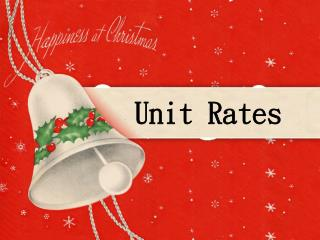Download PresentationUnit RatesUnit Rates - PowerPoint PPT Presentation

Download PresentationUnit Rates
An Image/Link below is provided (as is) to download presentation

Download Policy: Content on the Website is provided to you AS IS for your information and personal use and may not be sold / licensed / shared on other websites without getting consent from its author. While downloading, if for some reason you are not able to download a presentation, the publisher may have deleted the file from their server.

- - - - - - - - - - - - - - - - - - - - - - - - - - - E N D - - - - - - - - - - - - - - - - - - - - - - - - - - -
Presentation Transcript

1. Unit Rates

2. Unit Rates Rate– a ratio that compares different kinds of units by division to obtain a unit rateor rate per unit. (You are finding how much for 1 of something. Or the rate when the denominator is 1)

3. Unit Rates To express a ratio as a rate per unit, write the ratio as a fraction and then divide the numerator by the denominator. 395 miles in 5 hours miles miles 79 miles per hour = hours hour

4. Unit Rates 120 words in 3 minutes words 40 words per minute = per minute

5. Unit Rates If it costs \$78 for 13 sandwiches, What is the unit rate?

6. Unit Rates The cost of a 12-ounce box of Cheerios is \$3.29. Publix brand Cheerios cost \$4.89 for an 18-ounce box. Find the unit rate to find the better buy.

7. Unit Rates Miles per Gallon • M.P.G. stands for miles per gallon and is usually used for gas mileage in cars.

8. Unit Rates • If it takes 16 gallons to drive 352 miles, What is the unit rate or m.p.g.?

9. Unit Rates Pens can be purchased in a 5-pack for \$1.95 or a 15-pack for \$6.20. Which is the better buy? Divide the price by the number of pens. price for package number of pens \$1.95 5 =  \$0.39 price for package number of pens \$6.20 15 =  \$0.41 The better buy is the 5-pack for \$1.95.

10. price for jar number of ounces price for jar number of ounces Unit Rates Jamie can buy a 15-oz jar of peanut butter for \$2.19 or a 20-oz jar for \$2.78. Which is the better buy? Divide the price by the number of ounces. \$2.19 15 =  \$0.15 \$2.78 20 =  \$0.14 The better buy is the 20-oz jar for \$2.78.

11. Unit Rates 1. John can buy a 8 pencils for \$1.25 or a 12 pencils for \$2.00. Which is the better buy? \$1.25 / 8 = \$0.16 \$2.00 / 12 = \$0.17 2. Mary made \$25.00 for working 7 hours at the mall. How much did Mary make per hour? \$25.00 / 7 hours = \$3.57 / Hr

12. Unit Rates 4. Lisa paid \$158.00 for 3 pair of jeans. What did Lisa pay for each pair of jeans? \$158.00 / 3 pair of jeans = \$52.67 per jean 5. Bob typed 593 words in 4 minutes. How many words did Bob type per minute? 593 / 4 minutes = 148.3 words/minute